# From Signal to Symbols

23 de Nov de 2015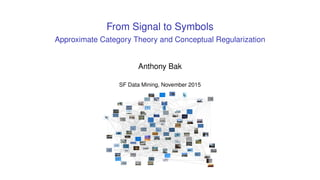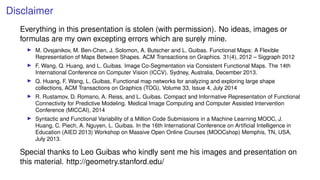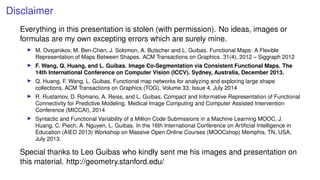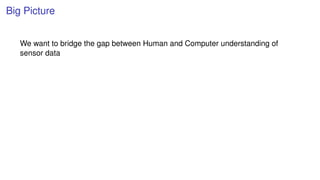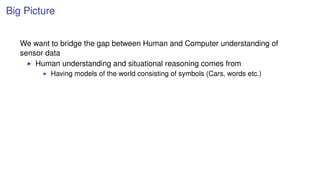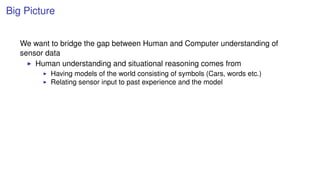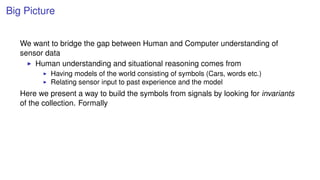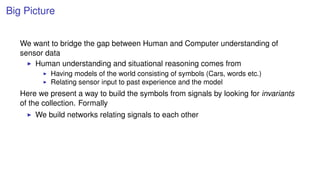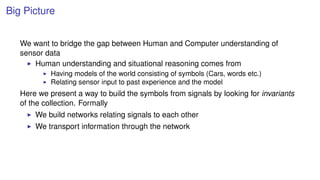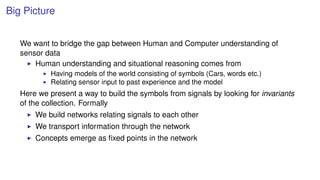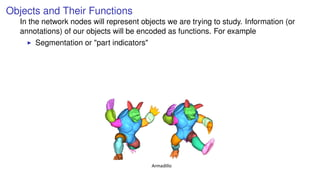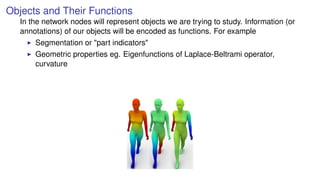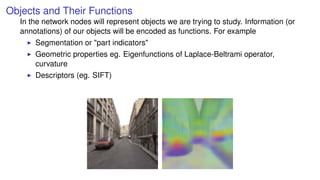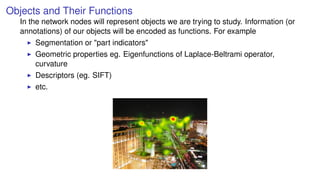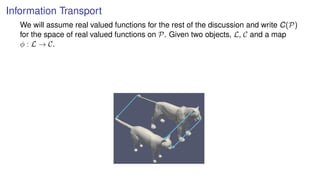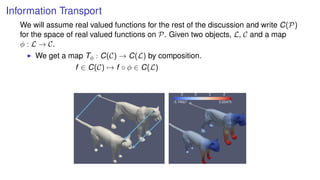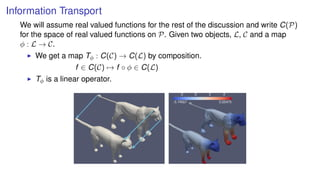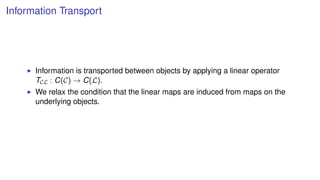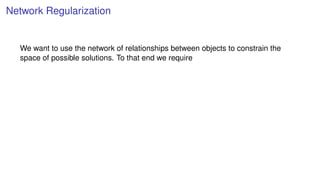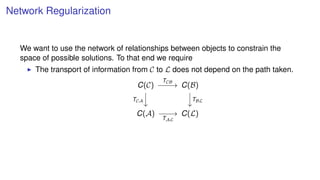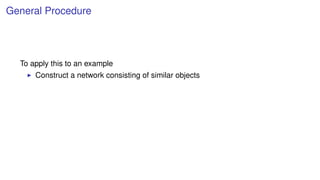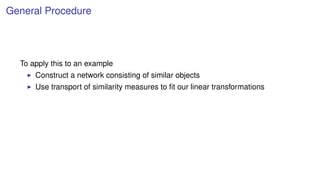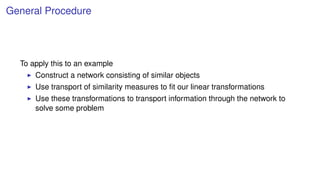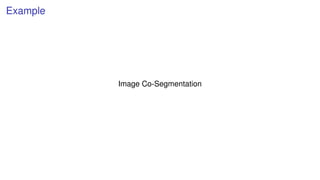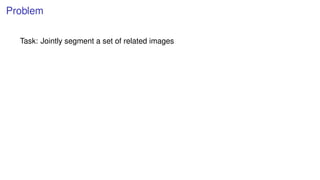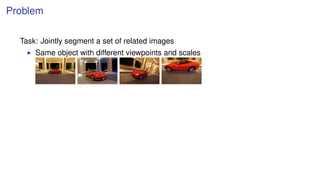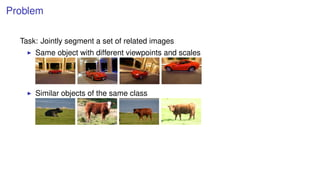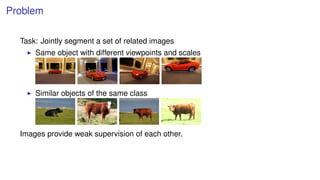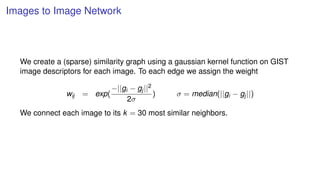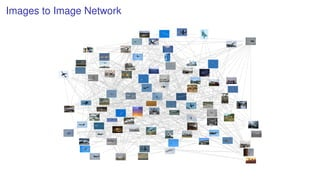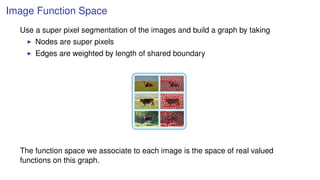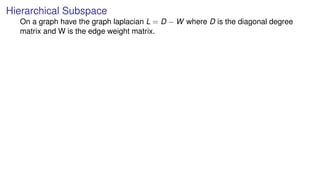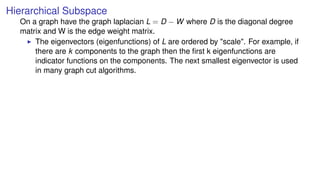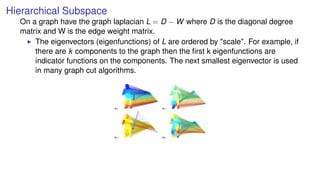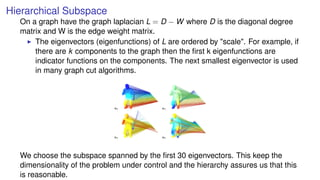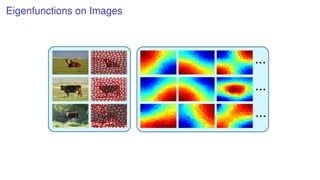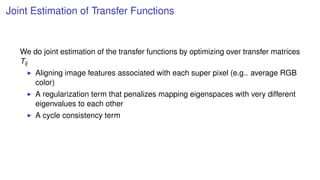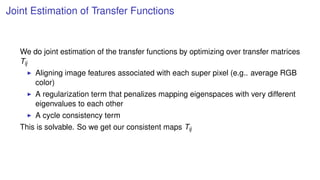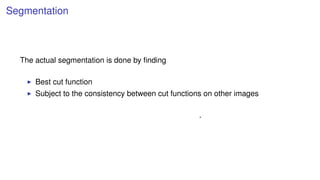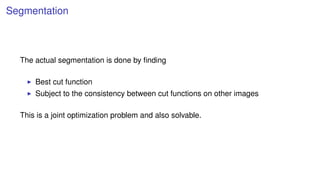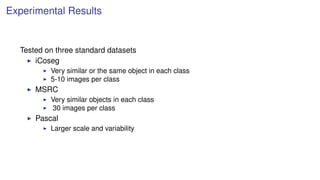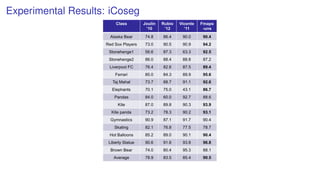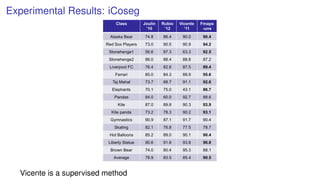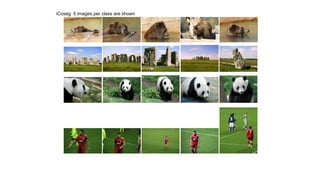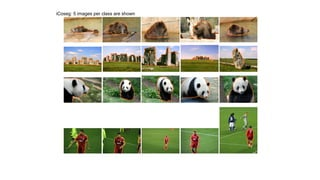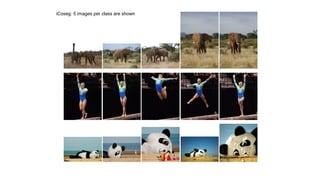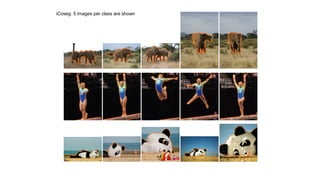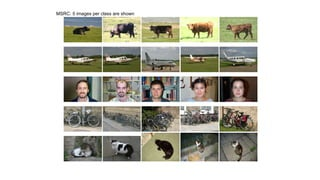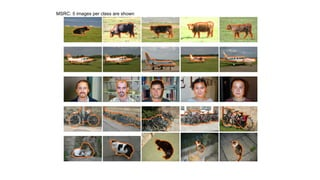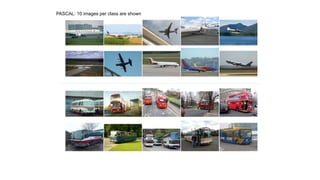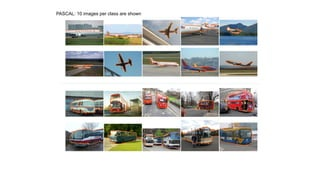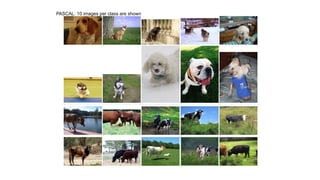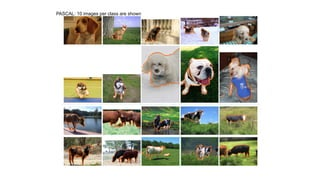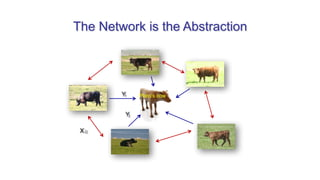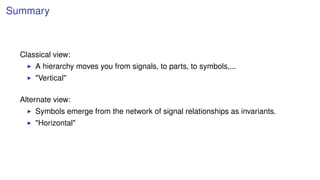1 de 56

### From Signal to Symbols

• 1. From Signal to Symbols Approximate Category Theory and Conceptual Regularization Anthony Bak SF Data Mining, November 2015
• 2. Disclaimer Everything in this presentation is stolen (with permission). No ideas, images or formulas are my own excepting errors which are surely mine. M. Ovsjanikov, M. Ben-Chen, J. Solomon, A. Butscher and L. Guibas. Functional Maps: A Flexible Representation of Maps Between Shapes. ACM Transactions on Graphics. 31(4), 2012 – Siggraph 2012 F. Wang, Q. Huang, and L. Guibas. Image Co-Segmentation via Consistent Functional Maps. The 14th International Conference on Computer Vision (ICCV). Sydney, Australia, December 2013. Q. Huang, F. Wang, L. Guibas, Functional map networks for analyzing and exploring large shape collections, ACM Transactions on Graphics (TOG), Volume 33, Issue 4, July 2014 R. Rustamov, D. Romano, A. Reiss, and L. Guibas. Compact and Informative Representation of Functional Connectivity for Predictive Modeling. Medical Image Computing and Computer Assisted Intervention Conference (MICCAI), 2014 Syntactic and Functional Variability of a Million Code Submissions in a Machine Learning MOOC, J. Huang, C. Piech, A. Nguyen, L. Guibas. In the 16th International Conference on Artiﬁcial Intelligence in Education (AIED 2013) Workshop on Massive Open Online Courses (MOOCshop) Memphis, TN, USA, July 2013. Special thanks to Leo Guibas who kindly sent me his images and presentation on this material. http://geometry.stanford.edu/
• 3. Disclaimer Everything in this presentation is stolen (with permission). No ideas, images or formulas are my own excepting errors which are surely mine. M. Ovsjanikov, M. Ben-Chen, J. Solomon, A. Butscher and L. Guibas. Functional Maps: A Flexible Representation of Maps Between Shapes. ACM Transactions on Graphics. 31(4), 2012 – Siggraph 2012 F. Wang, Q. Huang, and L. Guibas. Image Co-Segmentation via Consistent Functional Maps. The 14th International Conference on Computer Vision (ICCV). Sydney, Australia, December 2013. Q. Huang, F. Wang, L. Guibas, Functional map networks for analyzing and exploring large shape collections, ACM Transactions on Graphics (TOG), Volume 33, Issue 4, July 2014 R. Rustamov, D. Romano, A. Reiss, and L. Guibas. Compact and Informative Representation of Functional Connectivity for Predictive Modeling. Medical Image Computing and Computer Assisted Intervention Conference (MICCAI), 2014 Syntactic and Functional Variability of a Million Code Submissions in a Machine Learning MOOC, J. Huang, C. Piech, A. Nguyen, L. Guibas. In the 16th International Conference on Artiﬁcial Intelligence in Education (AIED 2013) Workshop on Massive Open Online Courses (MOOCshop) Memphis, TN, USA, July 2013. Special thanks to Leo Guibas who kindly sent me his images and presentation on this material. http://geometry.stanford.edu/
• 4. Big Picture We want to bridge the gap between Human and Computer understanding of sensor data
• 5. Big Picture We want to bridge the gap between Human and Computer understanding of sensor data Human understanding and situational reasoning comes from Having models of the world consisting of symbols (Cars, words etc.)
• 6. Big Picture We want to bridge the gap between Human and Computer understanding of sensor data Human understanding and situational reasoning comes from Having models of the world consisting of symbols (Cars, words etc.) Relating sensor input to past experience and the model
• 7. Big Picture We want to bridge the gap between Human and Computer understanding of sensor data Human understanding and situational reasoning comes from Having models of the world consisting of symbols (Cars, words etc.) Relating sensor input to past experience and the model Here we present a way to build the symbols from signals by looking for invariants of the collection. Formally
• 8. Big Picture We want to bridge the gap between Human and Computer understanding of sensor data Human understanding and situational reasoning comes from Having models of the world consisting of symbols (Cars, words etc.) Relating sensor input to past experience and the model Here we present a way to build the symbols from signals by looking for invariants of the collection. Formally We build networks relating signals to each other
• 9. Big Picture We want to bridge the gap between Human and Computer understanding of sensor data Human understanding and situational reasoning comes from Having models of the world consisting of symbols (Cars, words etc.) Relating sensor input to past experience and the model Here we present a way to build the symbols from signals by looking for invariants of the collection. Formally We build networks relating signals to each other We transport information through the network
• 10. Big Picture We want to bridge the gap between Human and Computer understanding of sensor data Human understanding and situational reasoning comes from Having models of the world consisting of symbols (Cars, words etc.) Relating sensor input to past experience and the model Here we present a way to build the symbols from signals by looking for invariants of the collection. Formally We build networks relating signals to each other We transport information through the network Concepts emerge as ﬁxed points in the network
• 11. Objects and Their Functions In the network nodes will represent objects we are trying to study. Information (or annotations) of our objects will be encoded as functions. For example Segmentation or "part indicators"
• 12. Objects and Their Functions In the network nodes will represent objects we are trying to study. Information (or annotations) of our objects will be encoded as functions. For example Segmentation or "part indicators" Geometric properties eg. Eigenfunctions of Laplace-Beltrami operator, curvature
• 13. Objects and Their Functions In the network nodes will represent objects we are trying to study. Information (or annotations) of our objects will be encoded as functions. For example Segmentation or "part indicators" Geometric properties eg. Eigenfunctions of Laplace-Beltrami operator, curvature Descriptors (eg. SIFT)
• 14. Objects and Their Functions In the network nodes will represent objects we are trying to study. Information (or annotations) of our objects will be encoded as functions. For example Segmentation or "part indicators" Geometric properties eg. Eigenfunctions of Laplace-Beltrami operator, curvature Descriptors (eg. SIFT) etc.
• 15. Information Transport We will assume real valued functions for the rest of the discussion and write C(P) for the space of real valued functions on P. Given two objects, L, C and a map φ : L → C.
• 16. Information Transport We will assume real valued functions for the rest of the discussion and write C(P) for the space of real valued functions on P. Given two objects, L, C and a map φ : L → C. We get a map Tφ : C(C) → C(L) by composition. f ∈ C(C) → f ◦ φ ∈ C(L)
• 17. Information Transport We will assume real valued functions for the rest of the discussion and write C(P) for the space of real valued functions on P. Given two objects, L, C and a map φ : L → C. We get a map Tφ : C(C) → C(L) by composition. f ∈ C(C) → f ◦ φ ∈ C(L) Tφ is a linear operator.
• 18. Information Transport Information is transported between objects by applying a linear operator TCL : C(C) → C(L). We relax the condition that the linear maps are induced from maps on the underlying objects.
• 19. Network Regularization We want to use the network of relationships between objects to constrain the space of possible solutions. To that end we require
• 20. Network Regularization We want to use the network of relationships between objects to constrain the space of possible solutions. To that end we require The transport of information from C to L does not depend on the path taken. C(C) C(B) C(A) C(L) TCB TCA TBL TAL
• 21. General Procedure To apply this to an example Construct a network consisting of similar objects
• 22. General Procedure To apply this to an example Construct a network consisting of similar objects Use transport of similarity measures to ﬁt our linear transformations
• 23. General Procedure To apply this to an example Construct a network consisting of similar objects Use transport of similarity measures to ﬁt our linear transformations Use these transformations to transport information through the network to solve some problem
• 25. Problem Task: Jointly segment a set of related images
• 26. Problem Task: Jointly segment a set of related images Same object with different viewpoints and scales
• 27. Problem Task: Jointly segment a set of related images Same object with different viewpoints and scales Similar objects of the same class
• 28. Problem Task: Jointly segment a set of related images Same object with different viewpoints and scales Similar objects of the same class Images provide weak supervision of each other.
• 29. Images to Image Network We create a (sparse) similarity graph using a gaussian kernel function on GIST image descriptors for each image. To each edge we assign the weight wij = exp( −||gi − gj||2 2σ ) σ = median(||gi − gj||) We connect each image to its k = 30 most similar neighbors.
• 30. Images to Image Network
• 31. Image Function Space Use a super pixel segmentation of the images and build a graph by taking Nodes are super pixels Edges are weighted by length of shared boundary The function space we associate to each image is the space of real valued functions on this graph.
• 32. Hierarchical Subspace On a graph have the graph laplacian L = D − W where D is the diagonal degree matrix and W is the edge weight matrix.
• 33. Hierarchical Subspace On a graph have the graph laplacian L = D − W where D is the diagonal degree matrix and W is the edge weight matrix. The eigenvectors (eigenfunctions) of L are ordered by "scale". For example, if there are k components to the graph then the ﬁrst k eigenfunctions are indicator functions on the components. The next smallest eigenvector is used in many graph cut algorithms.
• 34. Hierarchical Subspace On a graph have the graph laplacian L = D − W where D is the diagonal degree matrix and W is the edge weight matrix. The eigenvectors (eigenfunctions) of L are ordered by "scale". For example, if there are k components to the graph then the ﬁrst k eigenfunctions are indicator functions on the components. The next smallest eigenvector is used in many graph cut algorithms.
• 35. Hierarchical Subspace On a graph have the graph laplacian L = D − W where D is the diagonal degree matrix and W is the edge weight matrix. The eigenvectors (eigenfunctions) of L are ordered by "scale". For example, if there are k components to the graph then the ﬁrst k eigenfunctions are indicator functions on the components. The next smallest eigenvector is used in many graph cut algorithms.Examples of one-dimensional mappings u2 u3 u4 u8 Radu Horaud Graph Laplacian Tutorial
• 36. Hierarchical Subspace On a graph have the graph laplacian L = D − W where D is the diagonal degree matrix and W is the edge weight matrix. The eigenvectors (eigenfunctions) of L are ordered by "scale". For example, if there are k components to the graph then the ﬁrst k eigenfunctions are indicator functions on the components. The next smallest eigenvector is used in many graph cut algorithms.Examples of one-dimensional mappings u2 u3 u4 u8 Radu Horaud Graph Laplacian Tutorial We choose the subspace spanned by the ﬁrst 30 eigenvectors. This keep the dimensionality of the problem under control and the hierarchy assures us that this is reasonable.
• 38. Joint Estimation of Transfer Functions We do joint estimation of the transfer functions by optimizing over transfer matrices Tij Aligning image features associated with each super pixel (e.g.. average RGB color) A regularization term that penalizes mapping eigenspaces with very different eigenvalues to each other A cycle consistency term
• 39. Joint Estimation of Transfer Functions We do joint estimation of the transfer functions by optimizing over transfer matrices Tij Aligning image features associated with each super pixel (e.g.. average RGB color) A regularization term that penalizes mapping eigenspaces with very different eigenvalues to each other A cycle consistency term This is solvable. So we get our consistent maps Tij
• 40. Segmentation The actual segmentation is done by ﬁnding Best cut function Subject to the consistency between cut functions on other images .
• 41. Segmentation The actual segmentation is done by ﬁnding Best cut function Subject to the consistency between cut functions on other images This is a joint optimization problem and also solvable.
• 42. Experimental Results Tested on three standard datasets iCoseg Very similar or the same object in each class 5-10 images per class MSRC Very similar objects in each class 30 images per class Pascal Larger scale and variability
• 44. Experimental Results: iCoseg Vicente is a supervised method
• 45. iCoseg: 5 images per class are shown
• 46. iCoseg: 5 images per class are shown
• 47. iCoseg: 5 images per class are shown
• 48. iCoseg: 5 images per class are shown
• 49. MSRC: 5 images per class are shown
• 50. MSRC: 5 images per class are shown
• 51. PASCAL: 10 images per class are shown
• 52. PASCAL: 10 images per class are shown
• 53. PASCAL: 10 images per class are shown
• 54. PASCAL: 10 images per class are shown
• 55. The Network is the Abstraction Plato’s cow
• 56. Summary Classical view: A hierarchy moves you from signals, to parts, to symbols,... "Vertical" Alternate view: Symbols emerge from the network of signal relationships as invariants. "Horizontal"